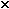# nonuniform circular motion q 1

1. A car speeds up as it turns from traveling due south to heading due east. When exactly halfway around the curve, the car’s acceleration is 1.8 m/s2, 23° north of east. What are the radial and tangential components of the acceleration at that point?

2. In the Bohr model of the hydrogen atom, an electron (mass =

9.1010
-31
kg) orbits a proton at a distance of

3.3910
-9
m. The proton pulls on the electron with an electric force of

2.0110
-11
N. How many revolutions per second does the electron make?

3. A highway curve of radius 410 m is designed for traffic moving at a speed of 90 km/hr. What is the correct banking angle of the road?